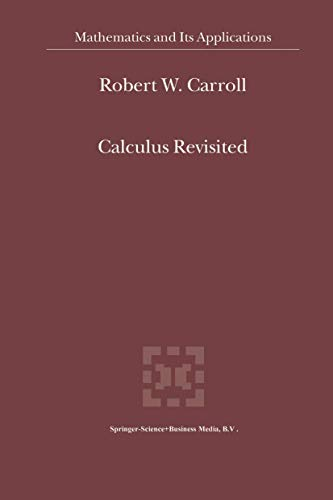• Free Shipping on all orders in Australia
• Over 7 million books in stock
• Proud to be B-Corp
• We aim to be carbon neutral by 2022
• Over 120,000 Trustpilot reviews
Item 1 of 0# Calculus Revisited by R.W. Carroll

Condition - New
\$388.09
Only 2 left

## Summary

In this book the details of many calculations are provided for access to work in quantum groups, algebraic differential calculus, noncommutative geometry, fuzzy physics, discrete geometry, gauge theory, quantum integrable systems, braiding, finite topological spaces, some aspects of geometry and quantum mechanics and gravity.

## Calculus Revisited Summary

### Calculus Revisited by R.W. Carroll

In this book the details of many calculations are provided for access to work in quantum groups, algebraic differential calculus, noncommutative geometry, fuzzy physics, discrete geometry, gauge theory, quantum integrable systems, braiding, finite topological spaces, some aspects of geometry and quantum mechanics and gravity.

Preface. 1. Basics for quantum groups. 2. Calculus and algebra. 3. Differential Calculi. 4. More on Q analysis. 5. Remarks on quantum transmutation. 6. Discretization and quantum mechanics. 7. Discrete calculus. 8. Fuzzy physics and matrix geometry. 9. Further aspects of FQM. 10. Integrable systems. 11. Aspects of gauge theory. 12. Pointless spaces and quantum gravity. Bibliography. Index

NLS9781441952370
9781441952370
1441952373
Calculus Revisited by R.W. Carroll
New
Paperback
Springer-Verlag New York Inc.
2010-12-07
512
N/A
Book picture is for illustrative purposes only, actual binding, cover or edition may vary.
This is a new book - be the first to read this copy. With untouched pages and a perfect binding, your brand new copy is ready to be opened for the first time